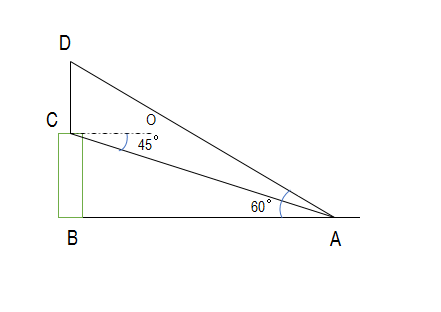QUESTION

# A pole 5m high is fixed on the top of a tower. The angle of elevation of the top of the poles observed from a point A on the ground is $60{}^\circ$ and the angle of depression of point A from the tower is $45{}^\circ$. Find the height of the tower. Take $\sqrt{3}=1.732$ .

Hint: The first thing you need to do is to draw a neat diagram. Then use the trigonometric ratios to find the height of the towers.

To start with the question, let us first draw a neat diagram of the situation given in the figure.Now it is given the question that CD = 5m, and we let CB to be h. Also, let AB to be b.

It is also given that $\angle DAB=60{}^\circ$ and $\angle ACO=45{}^\circ$ .

Also, it is clear from the diagram that $\angle BCO=90{}^\circ$ .

$\therefore \angle BCO=\angle ACO+\angle BCA=90{}^\circ$

Now if we put the value of $\angle ACO$ , we get

$45{}^\circ +\angle BCA=90{}^\circ$

$\Rightarrow \angle BCA=45{}^\circ$

Now let us focus on the right-angled triangle ADB.

We know $\tan \theta =\dfrac{perpendicular}{base}$ .So, we can say that: $\tan \left( \angle DAB \right)=\dfrac{BD}{AB}=\dfrac{h+5}{b}$ .

$\therefore \tan 60{}^\circ =\dfrac{h+5}{b}$

We also know that $\tan 60{}^\circ =\sqrt{3}$ , so putting the value in our equation, we get

$\sqrt{3}=\dfrac{h+5}{b}$

$\Rightarrow \sqrt{3}b=h+5$

$\Rightarrow b=\dfrac{h+5}{\sqrt{3}}$

Now we shall focus on the right-angled triangle ABC.

$\tan \left( \angle BCA \right)=\dfrac{AB}{CB}=\dfrac{b}{h}$

On putting $\angle BCA=45{}^\circ$, we get

$\tan \left( 45{}^\circ \right)=\dfrac{b}{h}$

We know that the value of $\tan 45{}^\circ$ is equal to 1. So, our equation becomes:

$1=\dfrac{b}{h}$

$\Rightarrow h=b$

Now we will substitute the value of b in terms of h from equation (i). On doing so, our equation becomes:

$h=\dfrac{h+5}{\sqrt{3}}$

$\sqrt{3}h-h=5$

As given in the question we put the value of $\sqrt{3}$ as 1.732, we get

$1.732h-h=5$

$\Rightarrow .732h=5$

$\Rightarrow h=\dfrac{5}{.732}=6.83$

So, we can conclude that the height of the tower is 6.83 m.

Note: While solving such questions, make sure that your diagram is correct and satisfies all the conditions given in the question, as the key to such questions is the diagram and the knowledge of simple trigonometric ratios.